# F-Test (ANCOVA)

The test statistic is: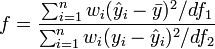$f = \frac{\sum^n_{i=1} w_i(\hat{y}_i - \bar{y})^2 / df_1}{\sum^n_{i=1} w_i(y_i - \hat{y}_i )^2 / df_2}$

where: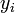$y_i$ is the$i$th of$n$ observed values of a numeric variable,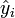$\hat{y}_i$ is a value fitted by weighted least squares,$df_1 = k$,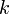$k$ is the number of independent variables in the weighted least squares (excluding the constant),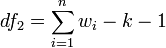$df_2 = \sum^n_{i=1}w_i - k - 1$,$w_i$ is the Calibrated Weight, and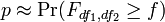$p \approx \Pr(F_{df_1,df_2} \ge f)$.